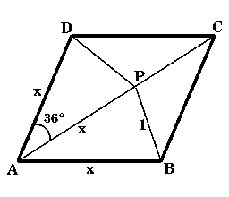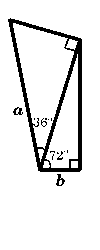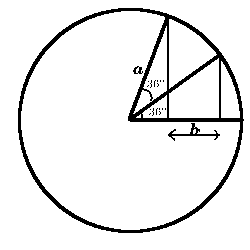#### You may also like### Pythagorean Golden Means

Show that the arithmetic mean, geometric mean and harmonic mean of a and b can be the lengths of the sides of a right-angles triangle if and only if a = bx^3, where x is the Golden Ratio.### Golden Triangle

Three triangles ABC, CBD and ABD (where D is a point on AC) are all isosceles. Find all the angles. Prove that the ratio of AB to BC is equal to the golden ratio.### Golden Powers

You add 1 to the golden ratio to get its square. How do you find higher powers?

# Gold Again

##### Age 16 to 18Challenge LevelConsider the rhombus as illustrated where $x$ is an unknown length, $AP = AD = x$ , angle $DAP = 36$ degrees and $P$ is a point on the diagonal $AC$ such that $PB = 1$ unit. Without using a calculator, computer or tables find the exact values of 1. $\cos36^{\circ}\cos72^{\circ}$ 2. $\cos36^{\circ} - \cos72^{\circ}.$3. Draw these two diagrams as accurately as you can and measure the lengths $a$ and $b$. What do you notice? Can you prove it? (In each diagram there are two right angled triangles).